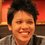# Evaluate this integral without using DogTeX

$\large \int_{0}^{\infty} \frac{e^{-x} \sin(x)\cos(x)}{\sqrt{x}}\,dx = \frac{\sqrt{\pi}}{2\cdot \sqrt{5}} \cdot \sin\left(\frac{1}{2} \tan^{-1}(2)\right)$

It is trivial to prove the equation above using DogTeX, but can you prove it without DogTeX?

This is a part of the set Formidable Series and IntegralsNote by Pi Han Goh
5 years, 3 months ago

This discussion board is a place to discuss our Daily Challenges and the math and science related to those challenges. Explanations are more than just a solution — they should explain the steps and thinking strategies that you used to obtain the solution. Comments should further the discussion of math and science.

When posting on Brilliant:

• Use the emojis to react to an explanation, whether you're congratulating a job well done , or just really confused .
• Ask specific questions about the challenge or the steps in somebody's explanation. Well-posed questions can add a lot to the discussion, but posting "I don't understand!" doesn't help anyone.
• Try to contribute something new to the discussion, whether it is an extension, generalization or other idea related to the challenge.

MarkdownAppears as
*italics* or _italics_ italics
**bold** or __bold__ bold
- bulleted- list
• bulleted
• list
1. numbered2. list
1. numbered
2. list
Note: you must add a full line of space before and after lists for them to show up correctly
paragraph 1paragraph 2

paragraph 1

paragraph 2

[example link](https://brilliant.org)example link
> This is a quote
This is a quote
    # I indented these lines
# 4 spaces, and now they show
# up as a code block.

print "hello world"
# I indented these lines
# 4 spaces, and now they show
# up as a code block.

print "hello world"
MathAppears as
Remember to wrap math in $$ ... $$ or $ ... $ to ensure proper formatting.
2 \times 3 $2 \times 3$
2^{34} $2^{34}$
a_{i-1} $a_{i-1}$
\frac{2}{3} $\frac{2}{3}$
\sqrt{2} $\sqrt{2}$
\sum_{i=1}^3 $\sum_{i=1}^3$
\sin \theta $\sin \theta$
\boxed{123} $\boxed{123}$

Sort by:

Let $\displaystyle \text{I} = \int_{0}^{\infty} \dfrac{e^{-x} \sin(x) \cos(x)}{\sqrt{x}} \mathrm{d}x$

$\displaystyle =\dfrac{1}{2} \int_{0}^{\infty} \dfrac{e^{-x} \sin(2x)}{\sqrt{x}} \mathrm{d}x$

$\displaystyle = \dfrac{1}{4i} \int_{0}^{\infty} \dfrac{e^{-x} (e^{2ix} - e^{-2ix})}{\sqrt{x}} \mathrm{d}x$

$\displaystyle = \dfrac{\text{A}-\text{A}^{*}}{4i}$

$\displaystyle = \dfrac{1}{2} \Im (\text{A})$

where $\displaystyle \text{A} = \int_{0}^{\infty} \dfrac{e^{-x(1-2i)}}{\sqrt{x}} \mathrm{d}x$

Note that,

$\displaystyle \Gamma (t) = \int_{0}^{\infty} x^{t-1} e^{-x} \mathrm{d}x$

Substituting $x \mapsto ax$, we have,

$\displaystyle \Gamma(t) = a^{t} \int_{0}^{\infty} x^{t-1} e^{-ax} \mathrm{d}x$

$\displaystyle \implies \text{A} = \Gamma \left( \dfrac{1}{2} \right) \dfrac{1}{\sqrt{1-2i}}$

$\displaystyle \implies \text{I} = \dfrac{\sqrt{\pi}}{2} \Im \left( \dfrac{1}{\sqrt{1-2i}} \right)$

$\displaystyle = \boxed{\dfrac{\sqrt{\pi}}{2 \sqrt{5}} \cdot \sin\left(\dfrac{1}{2} \tan^{-1}(2)\right)}$

- 5 years, 3 months ago

Wonderful work as usual! +1

- 5 years, 3 months ago

@Ishan Singh good ishu... :)

- 5 years, 3 months ago- 5 years, 3 months ago

So cute!!!!

- 5 years, 3 months ago

Marvellous solution! +1

- 5 years, 3 months ago

LOL!

- 5 years, 3 months ago